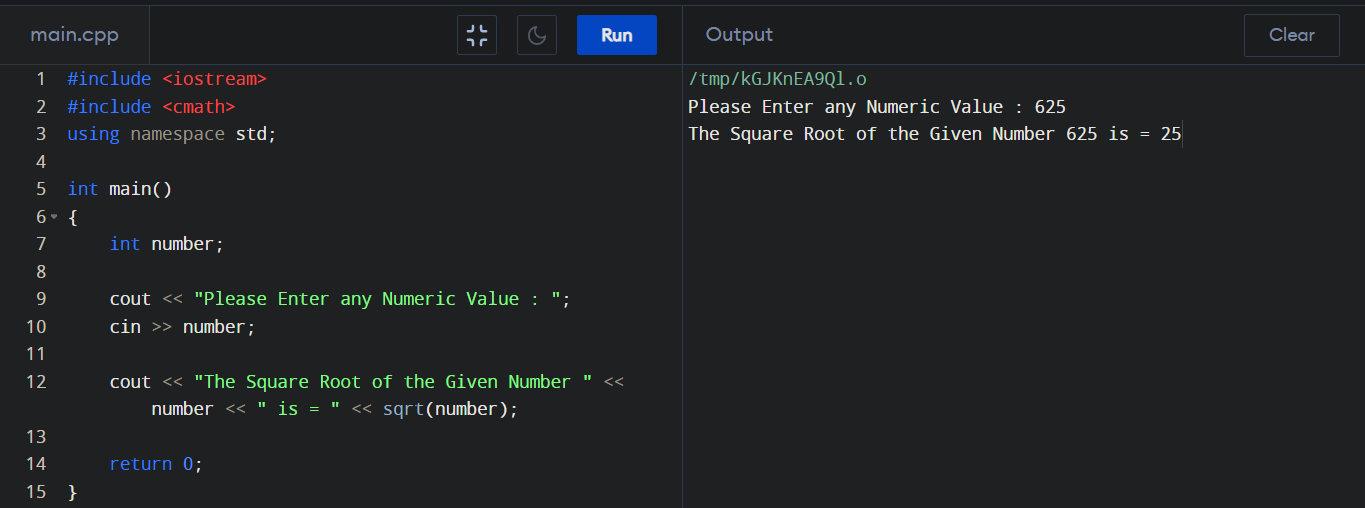C++ Program to Calculate Square Root of a Number

How to Calculate Square Root of a Number? We can do it by Dividing the Number with itself OR by Powering the Number by 1/2.

Square Root of a Number in C++ :

#include <iostream>
#include <cmath>
using namespace std;

int main()
{
int number;

cout << "Please Enter any Numeric Value : ";
cin >> number;

cout << "The Square Root of the Given Number " << number << " is = " << sqrt(number);

return 0;
}

Output :